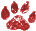# Validate An Algorithm With Another

 Namespace: WIN_COM_API
A useful practice in problem solving is to validate an algorithm with another.For example
see "How to solve it" by G. Polya, ISBN 069111966X.
In VFP we can use the #if ...#endif directive and the assert command to our advantage.
Put in a very simplistic way, we can exemplify this technique with the following code:
```set asserts on
ln_DataOne=5
ln_DataTwo=10
ln_ResultFirst=ln_DataOne*ln_DataTwo
#if file("develop.doc")
ln_Sum=0
for i=1 to ln_DataOne
ln_Sum=ln_Sum+ln_DataTwo
next
Assert ln_ResultFirst=ln_Sum Message "The algorithms have different results"
#endif
```

The use of file("Develop.doc") is to simulate a global variable and when develop.doc is
removed from the current directory the second algorithm is not executed.
Contributors Eduardo Díaz Guerrero
Category Code Samples
( Topic last updated: 2004.05.17 04:52:40 PM )